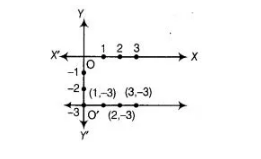# Draw the graph of the equation represented`
Question:

Draw the graph of the equation represented by a straight Line which is parallel to the X-axis and at a distance 3 units below it.

Solution:

Any straight line parallel to X-axis in negative direction of Y-axis is given by y = – k, where k is the distance of the line from the X-axis. Here, k = 3.Therefore, the equation of the line is y = -3. To draw the graph of this equation, plot the points (1,—3), (2, -3) and (3, -3) and join them. This is the required graph.